# Quiz 5: Supporting Facility and Process Flows

Follow the steps to calculate better layout design that would minimize the walking distance between different areas in the clinic as shown below: Step 1: Formulate the problem in excel as shown below: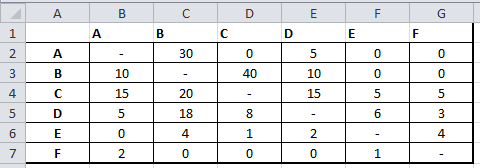Step 2: Triangularise the given matrix by adding the corresponding diagonal distance as shown below: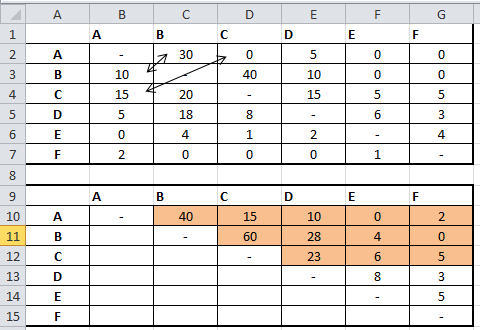Step 3: Formulate the initial layout as shown below: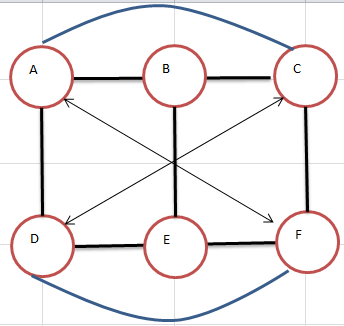Step 4: Calculate the non-adjacent pair loads as shown below: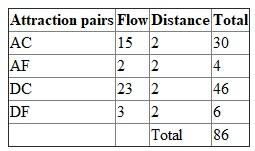Step 5: As the DC has the maximum load, therefore, move C close to D as shown below: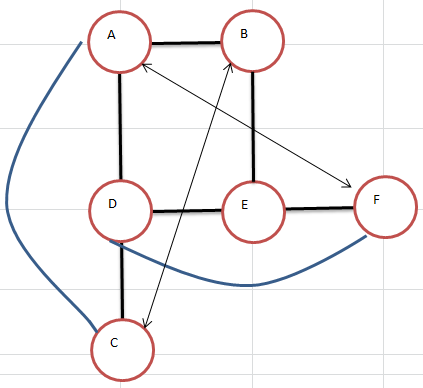Again, calculate the non-adjacent pair loads as shown below: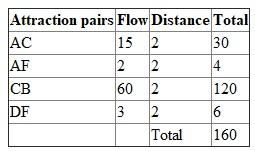As the CB has the maximum load, therefore, move B close to C as shown below: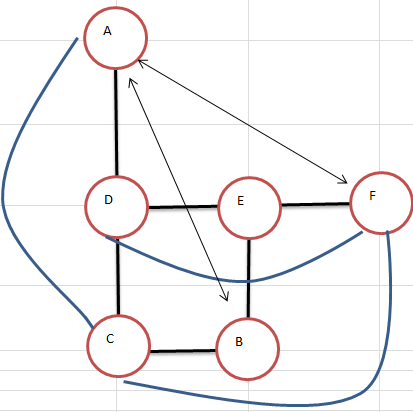Calculate the non-adjacent pair loads as shown below: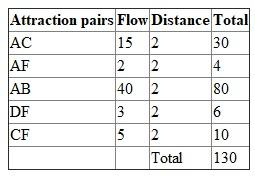As the AB has the maximum load, therefore, move B close to A as shown below: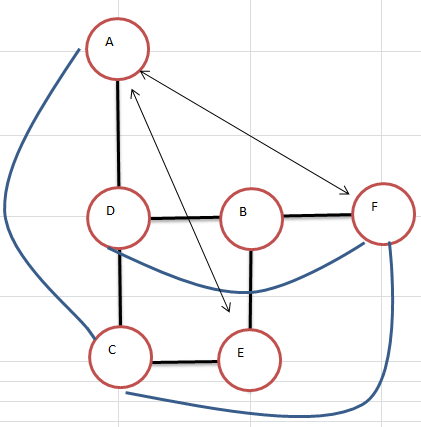Calculate the non-adjacent pair loads as shown below: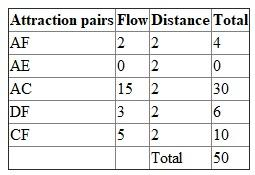AC has the maximum load, therefore, move C close to A as shown below: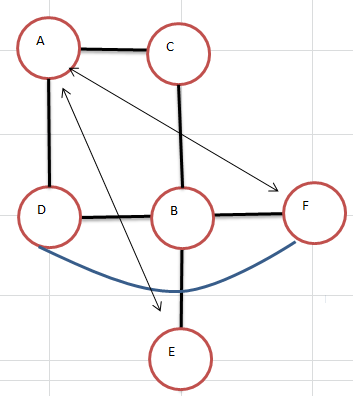Calculate the non-adjacent pair loads as shown below: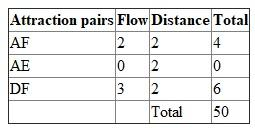As the DF has the maximum load, therefore, move F close to D as shown below: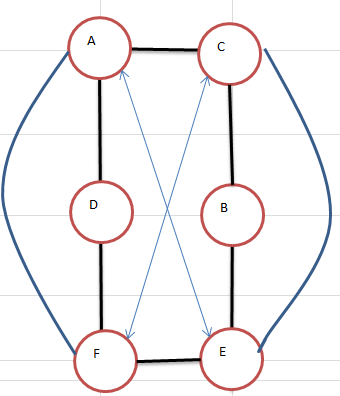Calculate the non-adjacent pair loads as shown below: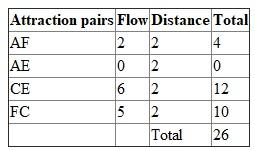The process will be stopped as all the areas of the clinic are now connected with its adjacent center. The minimum distance that one needs to travel by following this layout will be 26 meters
Activities 1.  Receive prescriptions 2.  Type labels 3.  Fill prescriptions 4.  Check prescriptions 5.  Dispense prescriptions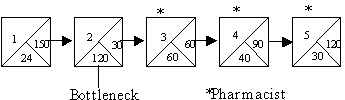Flow capacity is 30 patients/hour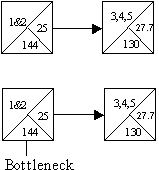Flow capacity is 50 patients/hour Staff:  2 clerks, 2 pharmacistsTriangularized matrix *Departments B and E cannot be adjacent Flow-Distance CalculationFinal layout